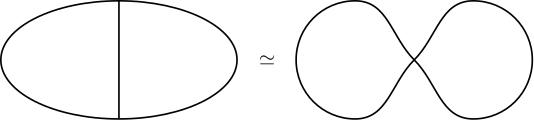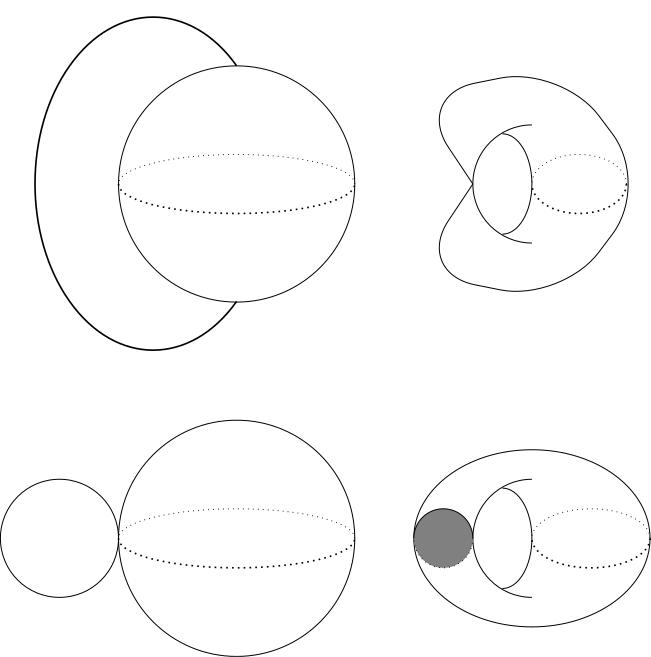# 4.02 Homotopy extension property (HEP)

Below the video you will find accompanying notes and some pre-class questions.

# Notes

(0.00) In this section, we will introduce the homotopy extension property, a useful tool for proving that different spaces are homotopy equivalent. For example, you can use it to prove that the \theta-graph and the figure 8 are homotopic just by squishing the middle bar of the \theta to a point'' (see image below), or the a sphere with a 1-cell attached (joining the North and South poles) is homotopy equivalent to a pinched torus just by squishing the 1-cell to the pinch point''.## Homotopy extension property

(0.58) Given a space $$X$$ and a subspace $$A$$, we say that the pair $$(X,A)$$ has the homotopy extension property (HEP) if, for every continuous map $$F\colon X\to Y$$ and every homotopy $$h\colon A\times[0,1]\to Y$$ with $$h(x,0)=F(x)$$ there exists a homotopy $$H\colon X\times[0,1]\to Y$$ such that $$H(x,0)=F(x)$$ and $$H|_{A\times[0,1]}=h$$.

(2.44) More colloquially, homotopies of functions defined on $$A$$ extend to homotopies of functions defined on $$X$$ (for given initial data).

(3.29) If $$(X,A)$$ has the HEP and $$A$$ is contractible, then $$X\simeq X/A$$, where $$X/A$$ denotes the quotient space in which $$A$$ is crushed to a single point.
(4.40) To prove that $$X\simeq X/A$$, we need to find continuous maps $$q\colon X\to X/A$$ and $$g\colon X/A\to X$$ such that $$g\circ q\simeq id_X$$ and $$q\circ g\simeq id_{X/A}$$. The map $$q\colon X\to X/A$$ will be the quotient map.

(5.20) Constructing the map $$g\colon X/A\to X$$. The subspace $$A$$ is contractible, so there exists a point $$a\in A$$ and a homotopy $$h_t\colon A\to A$$ such that $$h_0=id_A$$ and $$h_1(x)=a$$ for all $$x\in A$$. Since $$A\subset X$$, we can think of this as a homotopy $$h_t\colon A\to X$$.

(6.14) Using the HEP for the pair $$(X,A)$$, we get a homotopy $$H_t\colon X\to X$$ such that $$H_0=id_X$$ and $$(H_t)|_{A}=h_t$$. At $$t=1$$, $$h_1(A)=\{a\}$$ and $$(H_1)|_A=h_1$$, so $$H_1(A)=\{a\}$$. Therefore $$H_1=g\circ q$$ for some continuous map $$g\colon X/A\to X$$ (in other words, $$H_1$$ descends to the quotient).

(7.57) The fact that $$g$$ is continuous follows from this section on continuous maps out of a quotient space (continuous maps from $$X/\sim\to Y$$ are just continuous maps $$X\to Y$$ which descend to the quotient). This map $$g$$ is going to be our homotopy inverse for $$q$$.

(8.20) The map $$g$$ is a homotopy inverse for $$q$$. We need to prove:

1. (9.00) $$g\circ q\simeq id_X$$: This holds because $$g\circ q=H_1\simeq H_0=id_X$$.
2. (9.22) $$q\circ g\simeq id_{X/A}$$: Since $$(H_t)|_{A}=h_t$$, the homotopy $$q\circ H_t\colon X\to X/A$$ has the property that $$(q\circ H_t)(A)\subset q(h_t(A))\subset q(A)$$. Since $$q(A)$$ is a single point, this implies that $$q\circ H_t$$ factors as $$\bar{H}_t\circ q$$ for some continuous map $$\bar{H}_t\colon X/A\to X/A$$ (again, using our results on continuous maps out of a quotient space).

(11.22) We have $$\bar{H}_0=id_{X/A}$$ since $$H_0=id_X$$. We want to show that $$\bar{H}_1=q\circ g$$. We first notice that $\bar{H}_1\circ q=q\circ H_1=q\circ (g\circ q)=(q\circ g)\circ q,$ which implies $$\bar{H}_1=q\circ g$$ if we can cancel the extra $$q$$s on each side of the equation.

(12.34) We can cancel the $$q$$s because $$q$$ is surjective (it's a quotient map) and surjective maps have right-inverses (so can be cancelled from the right).

(14.05) We will see in the next video that if $$X$$ is a CW complex and $$A$$ is a closed subcomplex then $$(X,A)$$ has the HEP.

# Pre-class questions

1. Assuming that you can use the HEP with impunity, which of the following spaces are homotopy equivalent to one another?